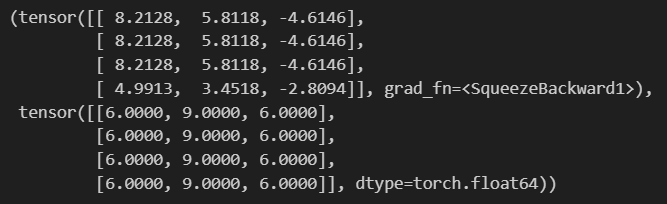# Output from nn.MultiheadAttention does not match calcs per Attention paper

Hi,
I’m trying to make sure I understand what nn.MultiheadAttention() is doing exactly. I have a very simple example below, where I would expect manual result and nn.MultiheadAttention() result to align but they do not. Is it normalization of the scores? Something else? What am I missing?

``````from torch.nn import functional as F
import torch
torch.set_printoptions(sci_mode=False)
torch.manual_seed(3)

# interaction embedding
mm=np.array([[1,1,1],[1,1,1],[1,1,1],[1,1,0]])
# random matrix for linear projection of mm
m=np.array([[2,3,1],
[2,3,2],
[2,3,3]])
# query, key and value matrix, all the same
q=np.dot(mm,m)

# manual calc
scores=q@q.transpose()
scores=torch.tensor(scores).float()
normalizer=q.shape**.05

# torch way
qt=torch.tensor(q).float()
builtin_attn_scores, my_attention_scores

``````

Output looks like thisOne thing that looks strange is that your implementation does not appear to do any projection to the input QKV matricies. Note that the PyTorch implementation applies projection matricies to each of the Q, K, and V: MultiheadAttention — PyTorch 2.0 documentation. I believe that the paper also does this, but it is kind of buried in the text (see the paragraph at the bottom of page 4).

You can check if using the weight matricies of the PyTorch layer in your manual calculation matches the results with the `q_proj_weight`, `k_proj_weight`, and `v_proj_weight` attributes.

Thank you for the response @eqy . My understanding of the projection is that it’s just a multiplication of the embedding matrix by some randomly initiated matrix. So in the above I thought of `mm` as an embedding and `m` as that random matrix. So np.dot(mm, m) should be equivalent to the linear projection. But now I realize also that my random matrix m, which is not even random :-), could be very different from the matrices initiated by the nn.MultiheadAttention() which would explain differences.

In hindsight I think it’s a silly question, of course they would be slightly different unless I made sure that all the matrices are the same. Appreciate you pointing that out.